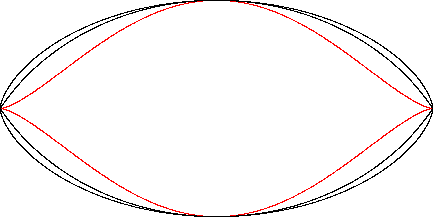Mouth Curve
MOUTH CURVE

Balmoral SoftwareThe "Mouth curve" is a bisymmetric sextic curve S with parametric equations
x(t) = cos(t)

y(t) = sin3(t), 0 ≤ t < 2π

The curve is traced out in a counterclockwise direction, starting from its right-hand cusp at (1,0). Its abscissa extrema are (±1,0) and its ordinate extrema (0,±1), so its bounding rectangle is a 2 x 2 square.

### Metrics

We have
x'(t) = -sin(t)

y'(t) = 3sin2(t)cos(t),

so by (L1), the perimeter of S is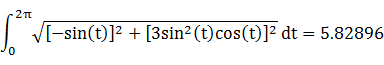By (A1), the area enclosed by S is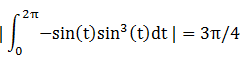### Convex Hull

We have
x''(t) = -cos(t)

y''(t) = 6sin(t) - 9sin3(t),

so by (X1), the curvature of S changes sign when t = 0 since that value is a solution to
-sin(t)[6sin(t) - 9sin3(t)] = -cos(t)[3sin2(t)cos(t)]
Equation (X2) is evaluated with respect to the point (x(0),y(0)) = (1,0) as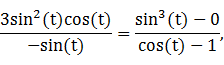which has a solution in the first quadrant at t = π/3. The convex hull is created by connecting the points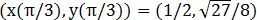and (1,0) with a straight line segment of length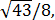and symmetrically in the other three quadrants. By (L1), the perimeter of the convex hull is: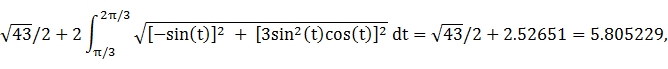which is about 0.4% shorter than the perimeter of S.

In the left figure below, the area of the Quadrant I portion of the triangle outlined in blue is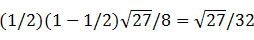and the area of the remaining convex hull area in Quadrant I is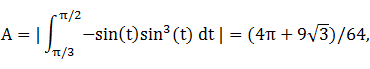so the total area of the convex hull is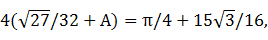which is about 2% larger than the area of S.

### Boundary Circles & Circumellipse

The squared distance function x2(t) + y2(t) ranges from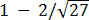to 1, so the inradius is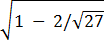and the circumradius is 1. Since the maximum distance of S from the origin along its positive and negative axes is the same, its circumellipse is the same as its circumcircle.

### Inellipse

From Lemma B,
x(t)y(t) = cos(t)sin3(t)
is maximized in the first quadrant when t* = π/3, so the inellipse dimensions are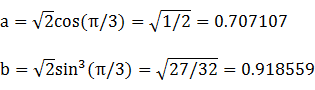For verification, we have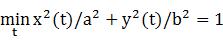### Summary Table

 Perimeter Area Centroid Figure Parameters Incircle R =4.927795 1.932393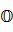Inellipse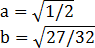5.128805 2.040524 Mouth curve Width: 2Height: 2 5.82896 2.356195 Convex hull 5.805229 2.409196 Circumcircle R = 1 6.283185 3.141593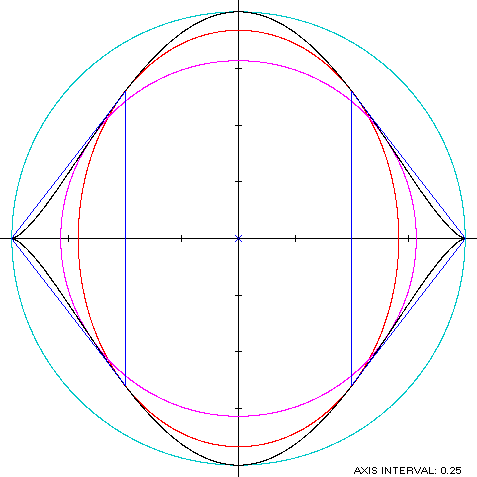The Aria

The mouth curve (red) is a member of a group of similarly-shaped figures described on these pages, including (inside to outside) the Vesica Piscis and the cycloid: